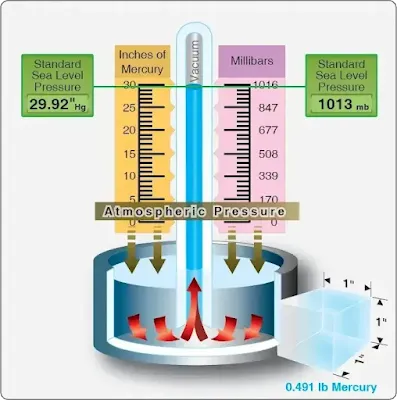# The Atmosphere

Before examining the fundamental laws of flight, several basic facts must be considered, namely that an aircraft operates in the air. Therefore, those properties of air that affect the control and performance of an aircraft must be understood.

The air in the earth’s atmosphere is composed mostly of nitrogen and oxygen. Air is considered a fluid because it fits the definition of a substance that has the ability to flow or assume the shape of the container in which it is enclosed. If the container is heated, pressure increases; if cooled, the pressure decreases. The weight of air is heaviest at sea level where it has been compressed by all of the air above. This compression of air is called atmospheric pressure.

## Pressure

Atmospheric pressure is usually defined as the force exerted against the earth’s surface by the weight of the air above that surface. Weight is force applied to an area that results in pressure. Force (F) equals area (A) times pressure (P), or F = AP. Therefore, to find the amount of pressure, divide area into force (P = F/A). A column of air (one square inch) extending from sea level to the top of the atmosphere weighs approximately 14.7 pounds; therefore, atmospheric pressure is stated in pounds per square inch (psi). Thus, atmospheric pressure at sea level is 14.7 psi.

Atmospheric pressure is measured with an instrument called a barometer, composed of mercury in a tube that records atmospheric pressure in inches of mercury ("Hg). [Figure] The standard measurement in aviation altimeters and U.S. weather reports has been "Hg. However, world-wide weather maps and some non-U.S. manufactured aircraft instruments indicate pressure in millibars (mb), a metric unit. At sea level, when the average atmospheric pressure is 14.7 psi, the barometric pressure is 29.92 "Hg, and the metric measurement is 1013.25 mb.Barometer used to measure atmospheric pressure

An important consideration is that atmospheric pressure varies with altitude. As an aircraft ascends, atmospheric pressure drops, oxygen content of the air decreases, and temperature drops. The changes in altitude affect an aircraft’s performance in such areas as lift and engine horsepower. The effects of temperature, altitude, and density of air on aircraft performance are covered in the following paragraphs.

## Density

Density is weight per unit of volume. Since air is a mixture of gases, it can be compressed. If the air in one container is under half as much pressure as an equal amount of air in an identical container, the air under the greater pressure weighs twice as much as that in the container under lower pressure. The air under greater pressure is twice as dense as that in the other container. For the equal weight of air, that which is under the greater pressure occupies only half the volume of that under half the pressure.

The density of gases is governed by the following rules:
1. Density varies in direct proportion with the pressure.
2. Density varies inversely with the temperature.

Thus, air at high altitudes is less dense than air at low altitudes, and a mass of hot air is less dense than a mass of cool air.

Changes in density affect the aerodynamic performance of aircraft with the same horsepower. An aircraft can fly faster at a high altitude where the density is low than at a low altitude where the density is greater. This is because air offers less resistance to the aircraft when it contains a smaller number of air particles per unit of volume.

## Humidity

Humidity is the amount of water vapor in the air. The maximum amount of water vapor that air can hold varies with the temperature. The higher the temperature of the air, the more water vapor it can absorb.
1. Absolute humidity is the weight of water vapor in a unit volume of air.
2. Relative humidity is the ratio, in percent, of the moisture actually in the air to the moisture it would hold if it were saturated at the same temperature and pressure.

Assuming that the temperature and pressure remain the same, the density of the air varies inversely with the humidity. On damp days, the air density is less than on dry days. For this reason, an aircraft requires a longer runway for takeoff on damp days than it does on dry days.

By itself, water vapor weighs approximately five-eighths as much as an equal amount of perfectly dry air. Therefore, when air contains water vapor, it is not as heavy as dry air containing no moisture.

RELATED POSTS